## Spherical Triangle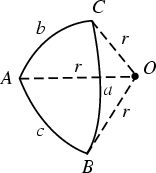A spherical triangle is a figure formed on the surface of a sphere by three great circular arcs intersecting pairwise in three vertices. The spherical triangle is the spherical analog of the planar Triangle. Let a spherical triangle have Angles,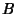, and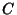and Radius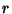. Then the Area of the spherical triangle is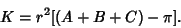The sum of the angles of a spherical triangle is between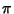and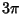radians (180° and 540°). The amount by which it exceeds 180° is called the Spherical Excess and is denoted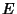or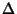.

The study of angles and distances of figures on a sphere is known as Spherical Trigonometry.

See also Colunar Triangle, Girard's Spherical Excess Formula, L'Huilier's Theorem, Spherical Polygon, Spherical Trigonometry

References

Abramowitz, M. and Stegun, C. A. (Eds.). Handbook of Mathematical Functions with Formulas, Graphs, and Mathematical Tables, 9th printing. New York: Dover, p. 79, 1972.

Beyer, W. H. CRC Standard Mathematical Tables, 28th ed. Boca Raton, FL: CRC Press, pp. 131 and 147-150, 1987.